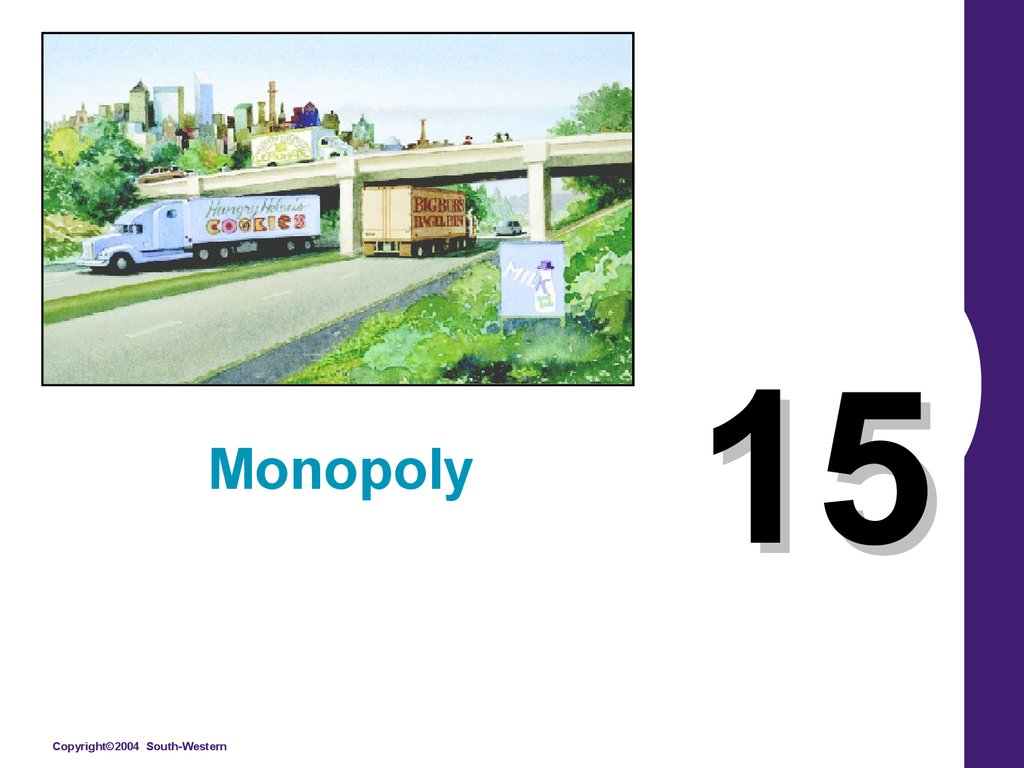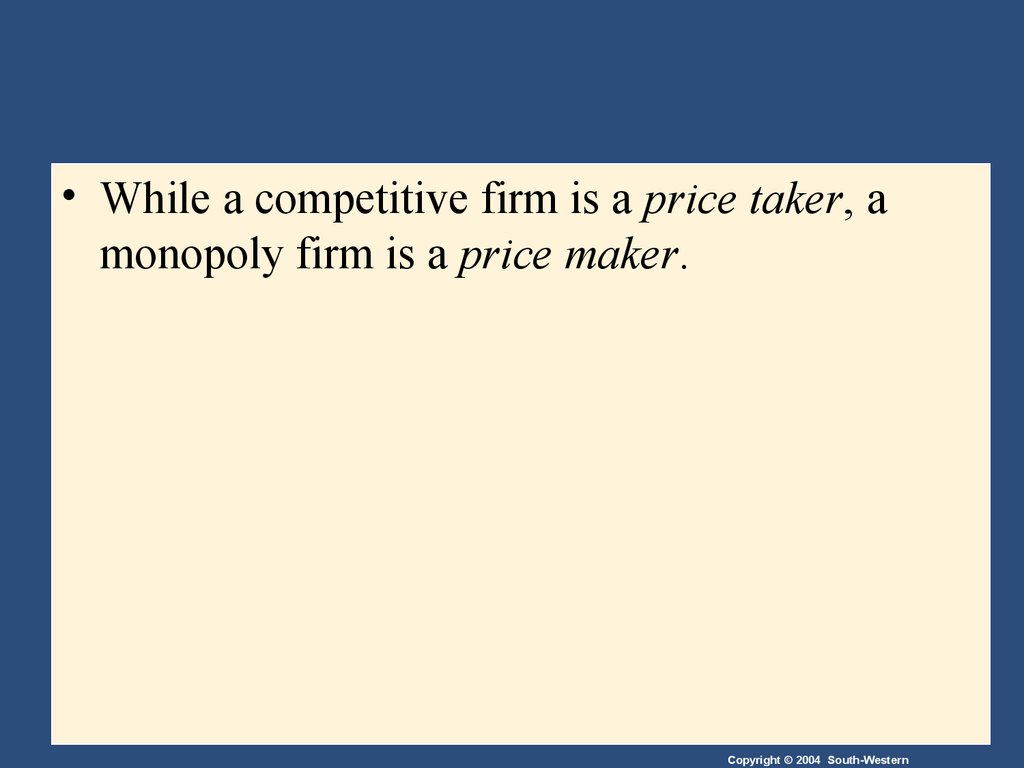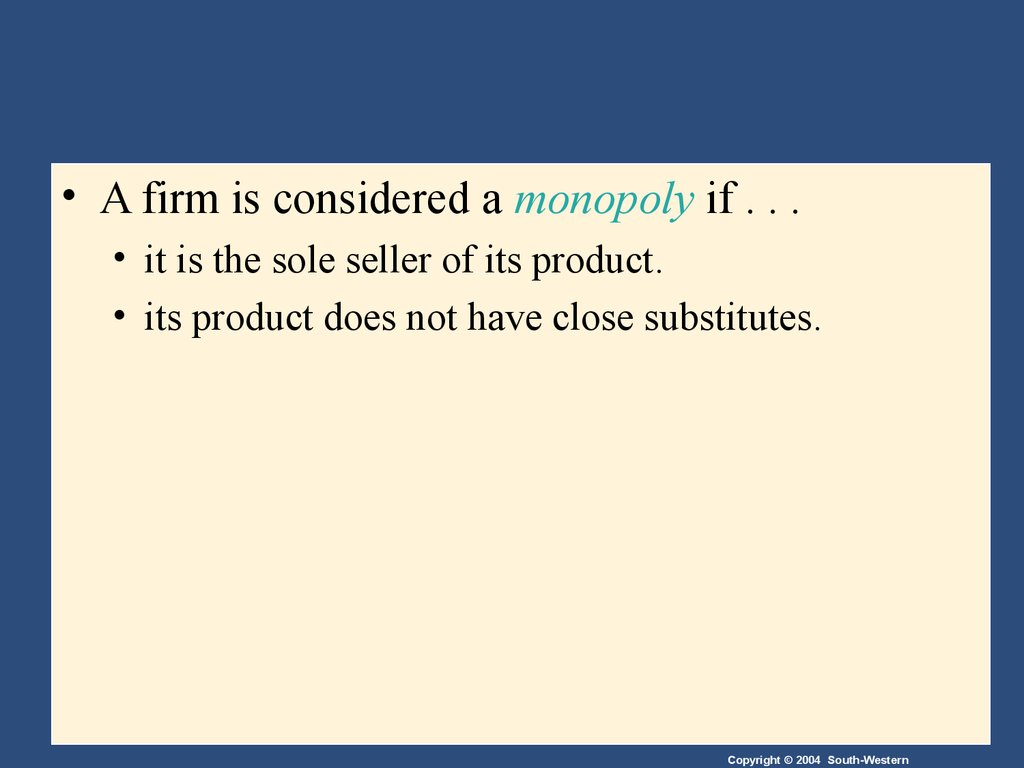# Monopoly. (Lecture 15)

Monopoly
15

## 2.

• While a competitive firm is a price taker, a
monopoly firm is a price maker.

## 3.

• A firm is considered a monopoly if . . .
• it is the sole seller of its product.
• its product does not have close substitutes.

## 4. WHY MONOPOLIES ARISE

• The fundamental cause of monopoly is barriers
to entry.

## 5. WHY MONOPOLIES ARISE

• Barriers to entry have three sources:
• Ownership of a key resource.
• The government gives a single firm the exclusive
right to produce some good.
• Costs of production make a single producer more
efficient than a large number of producers.

## 6. Monopoly Resources

• Although exclusive ownership of a key
resource is a potential source of monopoly, in
practice monopolies rarely arise for this reason.

## 7. Government-Created Monopolies

• Governments may restrict entry by giving a
single firm the exclusive right to sell a
particular good in certain markets.

## 8. Government-Created Monopolies

• Patent and copyright laws are two important
examples of how government creates a
monopoly to serve the public interest.

## 9. Natural Monopolies

• An industry is a natural monopoly when a
single firm can supply a good or service to an
entire market at a smaller cost than could two
or more firms.

## 10. Natural Monopolies

• A natural monopoly arises when there are
economies of scale over the relevant range of
output.

## 11. Figure 1 Economies of Scale as a Cause of Monopoly

Cost
Average
total
cost
0
Quantity of Output

## 12. HOW MONOPOLIES MAKE PRODUCTION AND PRICING DECISIONS

• Monopoly versus Competition
• Monopoly
Is the sole producer
Faces a downward-sloping demand curve
Is a price maker
Reduces price to increase sales
• Competitive Firm
Is one of many producers
Faces a horizontal demand curve
Is a price taker
Sells as much or as little at same price

## 13. Figure 2 Demand Curves for Competitive and Monopoly Firms

(a) A Competitive Firm’s Demand Curve
Price
(b) A Monopolist’s Demand Curve
Price
Demand
Demand
0
Quantity of Output
0
Quantity of Output

## 14. A Monopoly’s Revenue

• Total Revenue
P Q = TR
• Average Revenue
TR/Q = AR = P
• Marginal Revenue
TR/ Q = MR

## 16. A Monopoly’s Revenue

• A Monopoly’s Marginal Revenue
• A monopolist’s marginal revenue is always less
than the price of its good.
• The demand curve is downward sloping.
• When a monopoly drops the price to sell one more unit,
the revenue received from previously sold units also
decreases.

## 17. A Monopoly’s Revenue

• A Monopoly’s Marginal Revenue
• When a monopoly increases the amount it sells, it
has two effects on total revenue (P Q).
• The output effect—more output is sold, so Q is higher.
• The price effect—price falls, so P is lower.

## 18. Figure 3 Demand and Marginal-Revenue Curves for a Monopoly

Price
\$11
10
9
8
7
6
5
4
3
2
1
0
–1
–2
–3
–4
Demand
(average
revenue)
Marginal
revenue
1
2
3
4
5
6
7
8
Quantity of Water

## 19. Profit Maximization

• A monopoly maximizes profit by producing the
quantity at which marginal revenue equals
marginal cost.
• It then uses the demand curve to find the price
that will induce consumers to buy that quantity.

## 20. Figure 4 Profit Maximization for a Monopoly

Costs and
Revenue
2. . . . and then the demand
curve shows the price
consistent with this quantity.
B
Monopoly
price
1. The intersection of the
marginal-revenue curve
and the marginal-cost
curve determines the
profit-maximizing
quantity . . .
Average total cost
A
Demand
Marginal
cost
Marginal revenue
0
Q
QMAX
Q
Quantity

## 21. Profit Maximization

• Comparing Monopoly and Competition
• For a competitive firm, price equals marginal cost.
P = MR = MC
• For a monopoly firm, price exceeds marginal cost.
P > MR = MC

## 22. A Monopoly’s Profit

• Profit equals total revenue minus total costs.
• Profit = TR - TC
• Profit = (TR/Q - TC/Q) Q
• Profit = (P - ATC) Q

## 23. Figure 5 The Monopolist’s Profit

Costs and
Revenue
Marginal cost
Monopoly E
price
B
Monopoly
profit
Average
total D
cost
Average total cost
C
Demand
Marginal revenue
0
QMAX
Quantity

## 24. A Monopolist’s Profit

• The monopolist will receive economic profits
as long as price is greater than average total
cost.

Costs and
Revenue
Price
during
patent life
Price after
patent
expires
Marginal
cost
Marginal
revenue
0
Monopoly
quantity
Competitive
quantity
Demand
Quantity

## 26. THE WELFARE COST OF MONOPOLY

• In contrast to a competitive firm, the monopoly
charges a price above the marginal cost.
• From the standpoint of consumers, this high
price makes monopoly undesirable.
• However, from the standpoint of the owners of
the firm, the high price makes monopoly very
desirable.

## 27. Figure 7 The Efficient Level of Output

Price
Marginal cost
Value
to
Cost
to
monopolist
Value
to
Cost
to
monopolist
Demand
Quantity
0
is greater than
cost to seller.
is less than
cost to seller.
Efficient
quantity

• Because a monopoly sets its price above
marginal cost, it places a wedge between the
consumer’s willingness to pay and the
producer’s cost.
• This wedge causes the quantity sold to fall short of
the social optimum.

## 29. Figure 8 The Inefficiency of Monopoly

Price
loss
Marginal cost
Monopoly
price
Marginal
revenue
0
Monopoly Efficient
quantity quantity
Demand
Quantity

• The Inefficiency of Monopoly
• The monopolist produces less than the socially
efficient quantity of output.

• The deadweight loss caused by a monopoly is
similar to the deadweight loss caused by a tax.
• The difference between the two cases is that the
government gets the revenue from a tax,
whereas a private firm gets the monopoly
profit.

## 32. PUBLIC POLICY TOWARD MONOPOLIES

• Government responds to the problem of
monopoly in one of four ways.
• Making monopolized industries more competitive.
• Regulating the behavior of monopolies.
• Turning some private monopolies into public
enterprises.
• Doing nothing at all.

## 33. Increasing Competition with Antitrust Laws

• Antitrust laws are a collection of statutes aimed
at curbing monopoly power.
• Antitrust laws give government various ways to
promote competition.
• They allow government to prevent mergers.
• They allow government to break up companies.
• They prevent companies from performing activities
that make markets less competitive.

## 34. Increasing Competition with Antitrust Laws

• Two Important Antitrust Laws
• Sherman Antitrust Act (1890)
• Reduced the market power of the large and powerful
“trusts” of that time period.
• Clayton Act (1914)
• Strengthened the government’s powers and authorized
private lawsuits.

## 35. Regulation

• Government may regulate the prices that the
monopoly charges.
• The allocation of resources will be efficient if price
is set to equal marginal cost.

## 36. Figure 9 Marginal-Cost Pricing for a Natural Monopoly

Price
Average total
cost
Regulated
price
Loss
Average total cost
Marginal cost
Demand
0
Quantity

## 37. Regulation

• In practice, regulators will allow monopolists to
keep some of the benefits from lower costs in
the form of higher profit, a practice that
requires some departure from marginal-cost
pricing.

## 38. Public Ownership

• Rather than regulating a natural monopoly that
is run by a private firm, the government can run
the monopoly itself (e.g. in the United States,
the government runs the Postal Service).

## 39. Doing Nothing

• Government can do nothing at all if the market
failure is deemed small compared to the
imperfections of public policies.

## 40. PRICE DISCRIMINATION

• Price discrimination is the business practice of
selling the same good at different prices to
different customers, even though the costs for
producing for the two customers are the same.

## 41. PRICE DISCRIMINATION

• Price discrimination is not possible when a
good is sold in a competitive market since there
are many firms all selling at the market price.
In order to price discriminate, the firm must
have some market power.
• Perfect Price Discrimination
• Perfect price discrimination refers to the situation
when the monopolist knows exactly the willingness
to pay of each customer and can charge each
customer a different price.

## 42. PRICE DISCRIMINATION

• Two important effects of price discrimination:
• It can increase the monopolist’s profits.
• It can reduce deadweight loss.

## 43. Figure 10 Welfare with and without Price Discrimination

(a) Monopolist with Single Price
Price
Consumer
surplus
Monopoly
price
loss
Profit
Marginal cost
Marginal
revenue
0
Quantity sold
Demand
Quantity

## 44. Figure 10 Welfare with and without Price Discrimination

(b) Monopolist with Perfect Price Discrimination
Price
Profit
Marginal cost
Demand
0
Quantity sold
Quantity

## 45. PRICE DISCRIMINATION

• Examples of Price Discrimination
Movie tickets
Airline prices
Discount coupons
Financial aid
Quantity discounts

## 46. CONCLUSION: THE PREVALENCE OF MONOPOLY

• How prevalent are the problems of monopolies?
• Monopolies are common.
• Most firms have some control over their prices
because of differentiated products.
• Firms with substantial monopoly power are rare.
• Few goods are truly unique.

## 47. Summary

• A monopoly is a firm that is the sole seller in its
market.
• It faces a downward-sloping demand curve for
its product.
• A monopoly’s marginal revenue is always
below the price of its good.

## 48. Summary

• Like a competitive firm, a monopoly maximizes
profit by producing the quantity at which
marginal cost and marginal revenue are equal.
• Unlike a competitive firm, its price exceeds its
marginal revenue, so its price exceeds marginal
cost.

## 49. Summary

• A monopolist’s profit-maximizing level of
output is below the level that maximizes the
sum of consumer and producer surplus.
• A monopoly causes deadweight losses similar
to the deadweight losses caused by taxes.

## 50. Summary

• Policymakers can respond to the inefficiencies
of monopoly behavior with antitrust laws,
regulation of prices, or by turning the
monopoly into a government-run enterprise.
• If the market failure is deemed small,
policymakers may decide to do nothing at all.